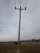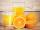# Poplar

How tall is a poplar by the river, if we know that 1/5 of its total height is a trunk, 1/10th of the height is the root and 35m from the trunk to the top of the poplar?

Result

x =  50 m

#### Solution:

35 + x/5+x/10 = x

7x = 350

x = 50

Calculated by our simple equation calculator.

Leave us a comment of example and its solution (i.e. if it is still somewhat unclear...):

Showing 0 comments:Be the first to comment!#### To solve this example are needed these knowledge from mathematics:

Need help calculate sum, simplify or multiply fractions? Try our fraction calculator. Do you have a linear equation or system of equations and looking for its solution? Or do you have quadratic equation? Do you want to convert length units?

## Next similar examples:

1. PlayingHow long have we trained on the pitch when we know that the warm-up took 10 minutes, we trained passes for one-third of the time and we played football half the time?
2. Unknown numberIdentify unknown number which 1/5 is 40 greater than one tenth of that number.
3. Sales storesThe first sales store passed the 1/3 and the second 2/5 of the total amount of goods.In third store passed the 2/3 rest of the goods. The remaining 40 kg of goods put into fourth store. How many kilograms of goods pass to a third store?
4. Unknown numberI think the number - its sixth is 3 smaller than its third.
5. Simple equationSolve for x: 3(x + 2) = x - 18
6. PearsThere were pears in the basket, I took two-fifths of them, and left six in the basket. How many pears did I take?
7. Equation with xSolve the following equation: 2x- (8x + 1) - (x + 2) / 5 = 9
8. EquationSolve the equation: 1/2-2/8 = 1/10; Write the result as a decimal number.
9. SeamstressThe seamstress cut the fabric into 3 parts. The first part was the eighth fabric, the second part was three-fifths of the fabric and the third part had a length of 66 cm. Calculate the original length of the fabric.
10. EqnSolve equation with fractions: 2x/3-50=40+x/4
11. Cleaning windowsCleaning company has to wash all the windows of the school. The first day washes one-sixth of the windows of the school, the next day three more windows than the first day and the remaining 18 windows washes on the third day. Calculate how many windows ha
12. FractionsThree-quarters of an unknown number are 4/5. What is 5/6 of this unknown number?
13. Simple equation 6Solve equation with one variable: X/2+X/3+X/4=X+4
14. Equation with fractionsSolve equation: ? It is equation with fractions.
15. UN 1If we add to an unknown number his quarter, we get 210. Identify unknown number.
16. Iron poleThe iron pole is in the ground 2/5 of its length, partly above the ground 1/3 is yellow, and the unpainted section is 6 m long. How long is the entire column?
17. TripOn the trip drank 3/10 of pupils tea, 2/5 cola, 1/4 mineral water and remaining 3 juice. How many students were on the trip?